# Balancing turning forces – the principle of momentsWhen an object is balanced on a pivot the turning effect of the forces on one side of the pivot must balance the turning effect of the forces on the other side of the pivot - if they didn't it would not balance.

In the picture (Figure 1(a) two girls are sitting on a see saw. They have moved until it is balanced. They are the same weight and so to balance the see saw they must sit the same distance from the pivot.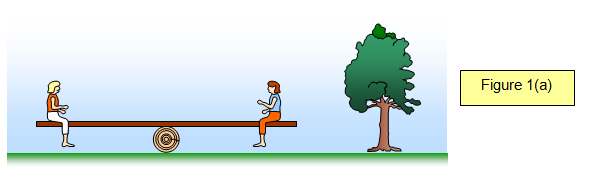In the picture (Figure 1(b) one of the girls gets off and a man sits on instead. They move until the see saw is balanced. The girl is much lighter than the man and so she has to sit further away from the pivot then he does so that she can balance his extra weight.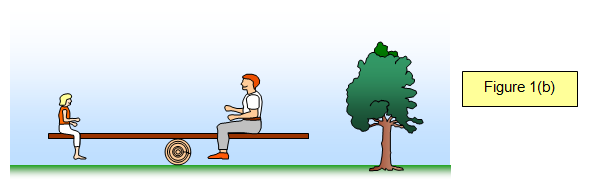You should remember that the turning effect of a force is called the moment of the force and is found by multiplying the force by its distance from the pivot. When the see saw is balanced we say that the anticlockwise moments (those trying to turn the object anticlockwise) equal the clockwise moments (those trying to turn the object clockwise). In our example the man's weight tries to turn the see saw clockwise and the girl's weight tries to turn it anticlockwise.

You can investigate this in the lab by using sets of weights hanging on a wooden ruler (Figure 2)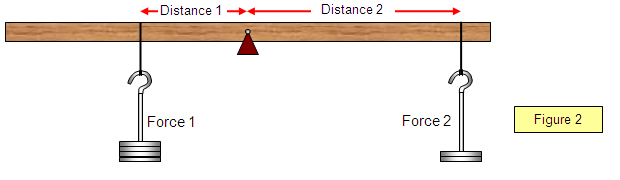The rule for something to be balanced is called the principle of moments and is written as follows:

The principle of moments

When an object is balanced (in equilibrium) the sum of the clockwise moments is equal to the sum of the anticlockwise moments.

Force 1 x distance 1 from pivot = Force 2 x distance 2 from pivot

F1xd1 = F2xd2

Example problem

Find the force needed to balance the lever.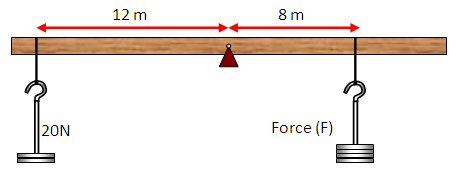Anticlockwise moment = 20 x 12 = 240 Nm
Clockwise moment = 8x F
8 x F = 240
F = 30 N

## A slightly more complicated version

The following diagram (Figure 3) shows the effect of having more than one force on each side of the pivot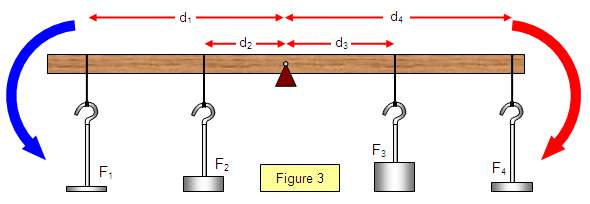In this case the balancing law becomes:

F1d1 + F2d2 = F3d3 + F4d4

A VERSION IN WORD IS AVAILABLE ON THE SCHOOLPHYSICS USB